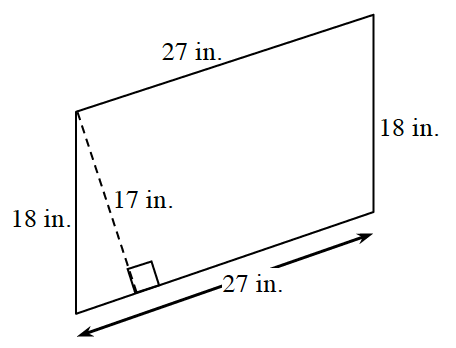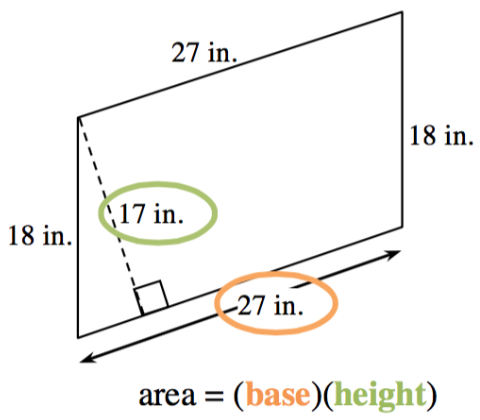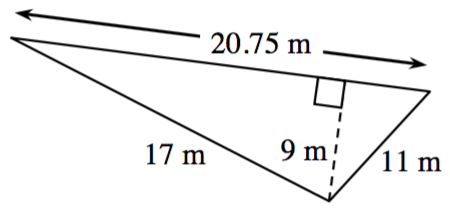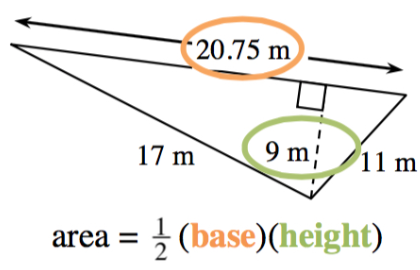Home > CC2 > Chapter 5 > Lesson 5.2.1 > Problem5-32

5-32.

Find the area of each figure below. Homework Help ✎

First, examine each shape to determine which type of shape it is. Look for characteristics such as right angles, same length sides, and number of sides.

After you have determined the type of shape, recall the area formulas specific to that shape. In this case, a parallelogram and a triangle.

1.$459$ sq. in.

1.